# Get Acquainted with Sample Charts in Excel With No Fee

If you have a task which concerns tables, charts, and functions and need help, you are at the right place. Our sample charts in Excel which you will see below will help you to cope with your assignment. This example was completed by an expert who is very knowledgeable in the topic. Look through the sample and try to follow the same steps as our expert to finish your task.

If your topic is similar to this one but still this sample is not what you need, you can read other samples on our blog or make an order. Sample charts in Excel are not the only type of example for solving assignments that we offer. On our blog, you can find many other examples in various topics. If you still need more help, you can order a specific example completed within your requirement from our experts. You can get samples in physics, biology, statistics, math, and other disciplines from AssignmentShark. Be quick to get help from us!

## Abstract

Temperature analysis

• Task 1: Organize Sheet 1, adding titles and formatting borders. The unknown column title is X.
• Task 2: Create a graph showing the contents of Columns A, D, F, and G against time.
• Task 3: Fill the yellow cells below using the appropriate formulas.
• Task 4: Include the diagram above and the graph in your report. Include a list of formulae used.

Electricity Production by Source

• Task 1: Observe the table included in Sheet2 representing the Electricity Production in China by the source in GhW 2008-2014.
• Task 2: Create a new table below the original one but in which the energy is displayed in TWh.
• Task 3: Complete the table by formatting its borders and, using Excel functions, calculate the total energy for each year.
• Task 4: Add a function showing the percentage of the total energy which comes from renewables.
• Task 5: Create a cumulative graph with the whole data, as shown below. Format the graph in a clear way.

## Introduction

The aim of this assignment is to improve my skills of working with tables, functions, and charts in Excel. It was a very interesting task; I’ve done a lot of research and improved my skills in Excel.

I will show my progress in a further report.

## Background Theory

Formatting tables in Excel

I used the tutorial which I found on the Microsoft support website. I have included it in my report:

There are various table styles in Excel. They are designed to give you the opportunity to save your time and format tables in Excel rapidly.

There is a possibility to create your own table styles if for some reason you want to change the ready-made tables.

You can delete or change your own styles, but the Excel ready-made table styles are not available for removal.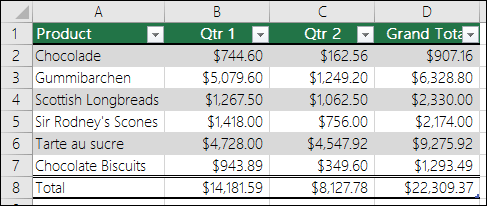Choosing the table style

Excel converts all the data into tables. If the document you are working with has some data which is not formatted, it will be converted to the table when you will choose one of the styles.

1. Select the range of data you want to convert into a table.
2. Select “Format as table” on the home menu in the upper part of the window.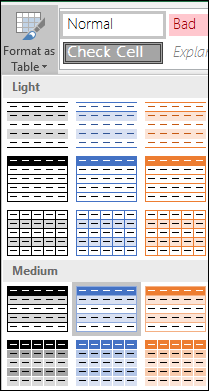3. Select the desired style.

Functions in Excel

I used the tutorial which I’ve found on the “excel-easy” website. I included it in my report:

The formula is an expression beginning with an equals sign and composed of numeric values, cell addresses, functions, and names, connected by the arithmetic operators. The arithmetic operators used in Excel are summation, subtraction, multiplication, division, and exponentiation.

Excel functions are special pre-created formulas for complex calculations that the user needs to enter only the arguments.

Functions consist of two parts: the function name and one or more arguments. The function name describes the operation that this function performs, for example, AMOUNTS.

The arguments of Excel functions set a value or a cell used by the function, and they are always enclosed in parentheses. An opening parenthesis without a space is put immediately after the function name. For example, in the formula “=SUM(A2;A9)”, SUM is the function name, and the A2 and A9 are its arguments.

Charts in Excel

I used the tutorial which I’ve found on the “excel-easy” website. I included it in my report:

To create a line chart, execute the following steps.

1. Select the range you want to use in your chart.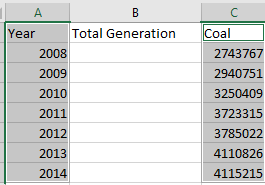2. On the Insert tab, in the Charts group, choose Line.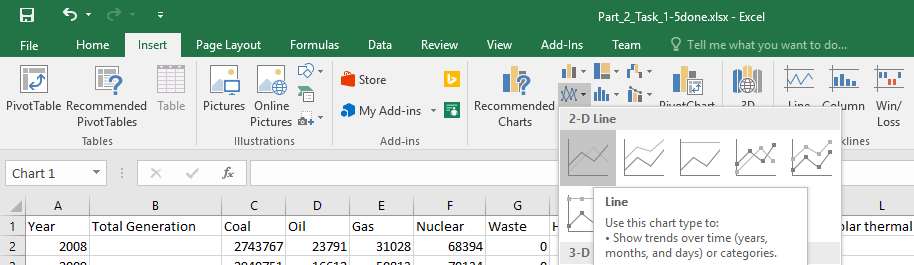## Experimental Procedure/Methodology

Temperature analysis

The first thing I completed was the formatting of the table. I selected all of the data in Excel spreadsheet and pushed the “Format as table” button on the “Home” menu at the top of the page.

Then I changed the names of the rows as it should be done in the task.

The second task was a graph showing the contents of Columns A, D, F, and G against time. I’ve selected these rows and pushed “2D line” on the graph bar in the “Insert” menu on the top of the page.

Then I filled the yellow cells as it was mentioned in the task. I used the “AVERAGE” function in Excel to count the mean value of the temperature and found the value of the difference between temperatures deducting the inlet values from the outlet values.

Then I used the mentioned formula (Q = ṁw * cp,w * ΔTc  (in Watts)) and counted the value of the heat transfer rate.

Electricity production by source

The first task was to create a new table below the original one but in which the energy is displayed in TWh. To do it I copied the original table and divided each value by 1000 (1 TWh = 1000Gwh).

Then I selected all of the data in Excel spreadsheet and pushed the “Format as table” button on the “Home” menu at the top of the page, and formatted the borders of cells.

I counted the total generation of electricity using the “SUM” Excel function. I filled each cell with its value.

I counted the percentage of the total energy that comes from renewables dividing the total value of electricity by the sum of the electricity which was generated by renewables, and formatted cells using the “Percentage” format.

Then I created a cumulative graph with all of the data as was shown in the task. I selected the required data, chose the “Recommended charts” and used the “Stacked area” chart.

Also, I created a pie graph of 2014. I selected the required data and used “Recommended charts” and chose the “Pie of pie” chart from all charts. I changed the amount of data in the additional pie from 5 to 8 to include all the renewables there as it was required in the task.

Temperature analysis

The first thing I completed was the formatting of the table. I selected all of the data in Excel spreadsheet and pushed the “Format as table” button on the “Home” menu at the top of the page.

Then I changed the names of the rows as it should be done in the task.

The second task was a graph showing the contents of Columns A, D, F, and G against time. I’ve selected these rows and pushed “2D line” on the graph bar in the “Insert” menu on the top of the page.

Then I filled the yellow cells as it was mentioned in the task. I used the “AVERAGE” function in Excel to count the mean value of the temperature and found the value of the difference between temperatures deducting the inlet values from the outlet values.

Then I used the mentioned formula (Q = ṁw * cp,w * ΔTc  (in Watts)) and counted the value of the heat transfer rate.

Electricity production by source

The first task was to create a new table below the original one but in which the energy is displayed in TWh. To do it I copied the original table and divided each value by 1000 (1 TWh = 1000Gwh).

Then I selected all of the data in Excel spreadsheet and pushed the “Format as table” button on the “Home” menu at the top of the page, and formatted the borders of cells.

I counted the total generation of electricity using the “SUM” Excel function. I filled each cell with its value.

I counted the percentage of the total energy that comes from renewables dividing the total value of electricity by the sum of the electricity which was generated by renewables, and formatted cells using the “Percentage” format.

Then I created a cumulative graph with all of the data as was shown in the task. I selected the required data, chose the “Recommended charts” and used the “Stacked area” chart.

Also, I created a pie graph of 2014. I selected the required data and used “Recommended charts” and chose the “Pie of pie” chart from all charts. I changed the amount of data in the additional pie from 5 to 8 to include all the renewables there as it was required in the task.

## Results and Analysis

Temperature analysis

I formatted the table as it was required in the task and received the following results: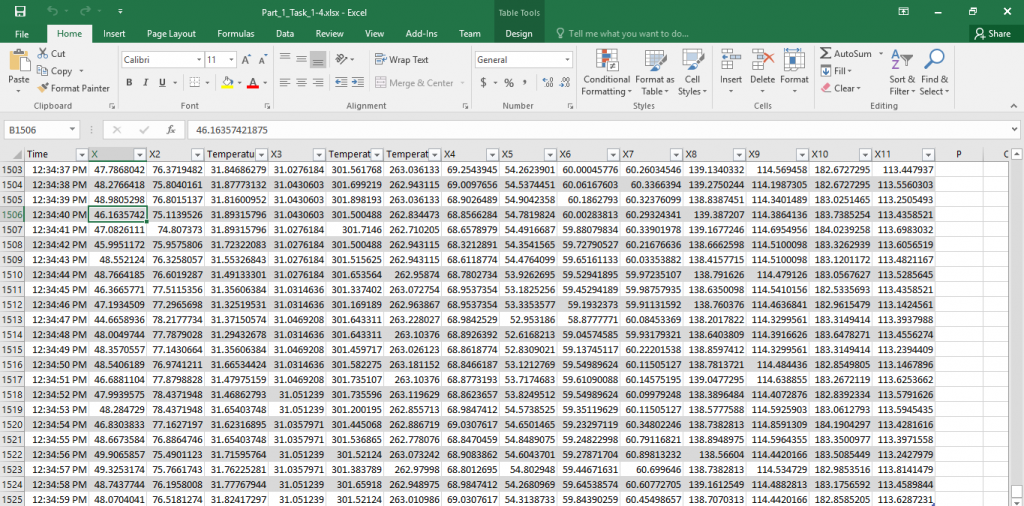I created a required chart and received the following result: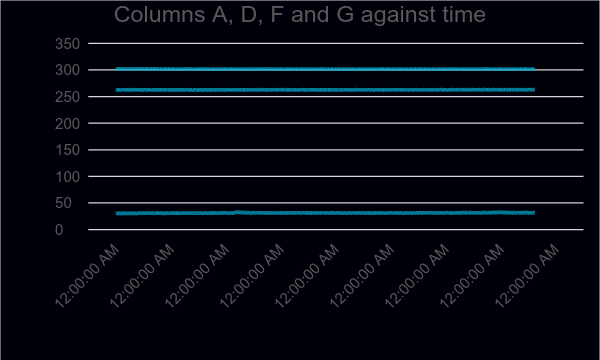Electricity production by source

I formatted the table as it was required in the task and received the following results: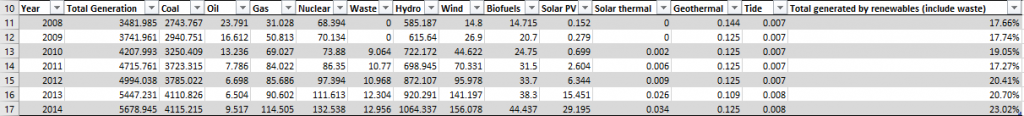I created the required charts and got the following result: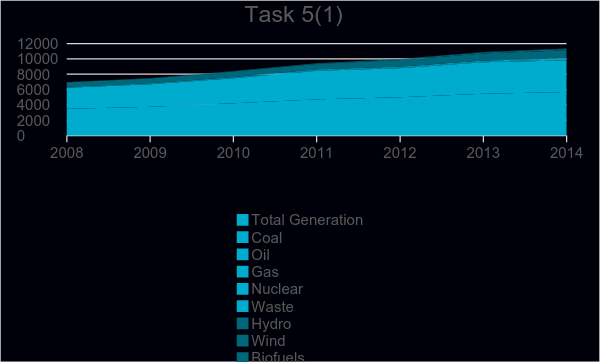## Discussion of Results

In this task I faced the problems of formatting tables, using functions, and graphing in Excel. It was a very interesting experience. I realized the power of a technological tool such as Excel, and understood how much it helps accountants and analysts to work with data, to visualize and analyze it. I really enjoyed doing this assignment.

## Conclusions and Recommendations

This task was a great opportunity to improve my skills in Excel. It is not too complicated, but still, it requires research and analysis. I have no comments on this assignment as in my opinion it is designed perfectly.